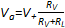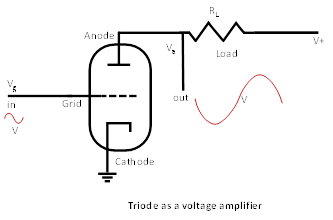Figure 6 shows a very common configuration where a resistor (loadRL is placed between the power supply V+ and the anode. The load and the resistance presented by the vacuum tube itself form a voltage divider.

This configuration composes a basic voltage amplifier, where the output signal is taken between the anode and the load. In fact, when the voltage grid varies, the current traversing the vacuum tube varies as well, because of the variation of the vacuum tube resistance. Consequently, the voltage measured between the anode and the cathode, called the anode voltage or the plate voltage, changes according to the voltage divider equation:,

where Va is the anode voltage, Rv, is the resistance offered by the vacuum tube, regulated by the grid voltage, and RL is the load resistance. In an appropriately designed voltage amplifier, small grid voltage variations ΔVg produce much larger anode voltage variations ΔVa.Figure 6: Vacuum tube as a voltage amplifier.A vacuum tube can be used as a voltage amplifier by placing a load between the anode power supply and the anode itself. The load forms a voltage divider with the resistance offered by the vacuum tube, which can be controlled by varying the grid voltage. The voltage signal applied to the grid is amplified by the voltage signal seen at the anode.

Note that the amplified voltage signal is inverted with respect to the signal voltage applied to the grid. In fact, when the signal voltage increases, the current from anode to cathode also increases, that is the resistance offered by the vacuum tube decreases, and correspondingly the voltage seen at the anode decreases as well.

Amplifiers with a large voltage gain, and limited current variation, are voltage amplifiers. They are generally used to increase the input signal before passing it to a power amplifier.

Amplifiers offering also a significant current variation are power amplifiers. They are generally used where high power is required across the load. In this case, the load is not just used to form a voltage divider with the vacuum tube. The load is the component that actually makes use of the amplified power. In power amplifiers built using vacuum tubes, as discussed later, generally the load consists of an output transformer, which adapts the impedance of the speaker to the load required by the vacuum tube.

In case of vacuum tubes used in input stages, as for instance 12AX7 vacuum tubes, given that the current variation from the anode to the cathode is very small, only voltage amplification is generally exploited.

In case of an output/power vacuum tube, as for instance EL34 tubes, given that significant current variation occurs from the anode to the cathode, we have a power amplifier.